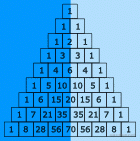# 3周攻克数据结构[数组篇-3]

## DAY4

### 1. 重塑矩阵

``````在MATLAB中，有一个非常有用的函数 reshape，它可以将一个矩阵重塑为另一个大小不同的新矩阵，但保留其原始数据。

nums =
[[1,2],
[3,4]]
r = 1, c = 4

[[1,2,3,4]]

nums =
[[1,2],
[3,4]]
r = 2, c = 4

[[1,2],
[3,4]]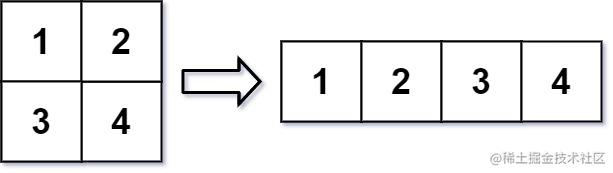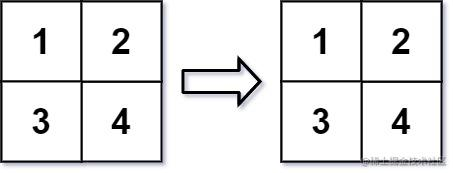#### 方法1：双循环

1. 拍平数组
2. 按照行数`r`，列数`c` 重新排列
``````function matrixReshape(mat: number[][], r: number, c: number): number[][] {
// 拍平二维数组
let newMat: any = mat.flat()

// 判断个数是否一致，一致才能重塑
if (r * c !== newMat.length) return mat

// r 行
for (let i = 0; i < r; i++) {
const item: number[] = []
// 每行c个
for (let j = 0; j < c; j ++){
// 将c个元素从头部拿出，并放入暂存的item数组
item.push(newMat.shift(newMat[i]))
}
// 当前行收集完毕，推入新数组的尾部
newMat.push(item);
}

return newMat
};

#### 方法2：二维数组一维表示

``````function matrixReshape(mat: number[][], r: number, c: number): number[][] {
const m = mat.length;
const n = mat.length;
if (m * n != r * c) {
return mat;
}

const ans = new Array(r).fill(0).map(() => new Array(c).fill(0));
for (let x = 0; x < m * n; ++x) {
ans[Math.floor(x / c)][x % c] = mat[Math.floor(x / n)][x % n];
}
return ans;
};

### 2. 杨辉三角

``````给定一个非负整数 numRows，生成杨辉三角的前 numRows 行。

[
,
[1,1],
[1,2,1],
[1,3,3,1],
[1,4,6,4,1]
]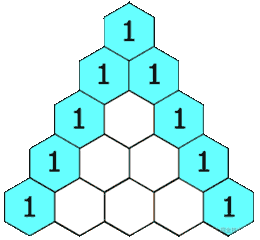#### 方法1：2层遍历求解

1. 每行的第一个元素和最后一个元素永远不会是上一层的和，因为他永远都是1
2. 下一层的值(x),位置(y) 取决上一层的和

``````x = 当前值
y = 层数
z = 位置

x = y - 1 [ z -1 ] + y - 1 [ z + 1 ]

``````function generate(numRows: number): number[][] {
const ret = []
for(let i = 0; i < numRows; i++) {
// 创建行，用 1 填充
const row = new Array(i + 1).fill(1);
// 填充每一行数据，左右2边不填充, 从 1 开始，结束于倒数第二个
for (let j = 1; j < row.length - 1; j++){
// 当前的值 = (上一行的当前个 - 1 [上一个] 值  ) + ( 上一行的当前个数 [下一个] 值 )
row[j] = ret[i - 1][j - 1] + ret[i - 1][j];
}
// 添加当前行
ret.push(row);
}

return ret;
};

### 科普篇：杨辉三角

``````要想画杨辉三角，先把 "1" 方在顶点，然后连续在下面按三角形的模式放上数字。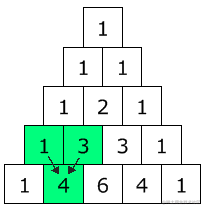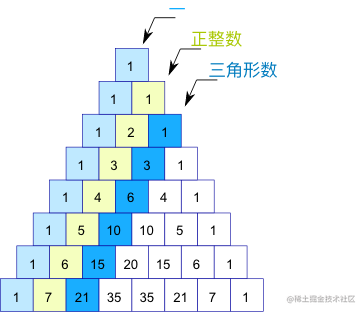``````第一条对角线全是 "1"